Definitions of Square Dance Calls and Concepts
Diagonal Column | Line | Wave Concept [C4]Index -->  Plus  |  A1  |  A2  |  C1  |  C2  |  C3A  |  C3B  |  C4  |  NOL  |
Definitions (Text Only) -->  Plus  |  A1  |  A2  |  C1  |  C2  |  C3A  |  C3B  |  C4  |  NOL  |
 Find call:

Diagonal Column | Line | Wave Concept -- [C4]

 \$B8@8l(B

A Diagonal Column | Line | Wave is a Distorted Column | Line | Wave in which the facing directions of the dancers are off by 45° relative to the Column | Line | Wave (i.e., if everybody individually turned 45° to the Right or Left, then they would be in the named formation). For example, from a Butterfly formation, there are two Diagonal Columns or two Diagonal Lines | Waves.

Diagonal Column: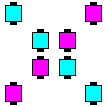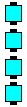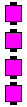\$B\$3\$3\$+\$i(B ... Think of working here... or here.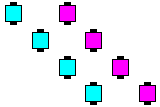These are (Twin) Diagonal Columns. You can work in a single Column or in Twin Columns depending upon the call.

Diagonal Column Dixie Style To A Wave: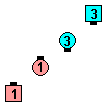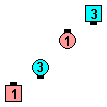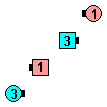Diagonal Column Dixie Style To A Wave\$B\$NA0(B Centers Right Pull By\$B\$N8e(B Left Touch 1/4\$B\$N8e(B (\$B=*\$o\$j(B)

Diagonal Line | Wave: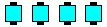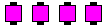\$B\$3\$3\$+\$i(B ... Think of working here... or here.

Normally, calls done within a Diagonal Column|Line|Wave start and end on the four footprints of the Diagonal Column|Line|Wave. Occasionally, from a Butterfly, the caller may give a call which rotates the Diagonal by 90° (such as Diagonal Wave Lockit or Diagonal Wave Spin The Top). In this case, the dancers end in the footprints of the other 1x4.

Diagonal Wave Spin The Top: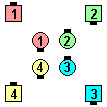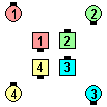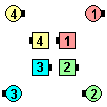Diagonal Wave Spin The Top\$B\$NA0(B Diagonal Wave Swing\$B\$N8e(B Diagonal Wave Fan The Top\$B\$N8e(B (\$B=*\$o\$j(B)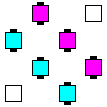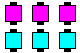From Interlocked Blocksyou have Diagonal Lines of 3... Think of working here.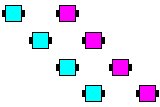These are (Parallel) Diagonal Lines. You can work in a single Line or in Parallel Lines depending upon the call.Comments? Questions? Suggestions?